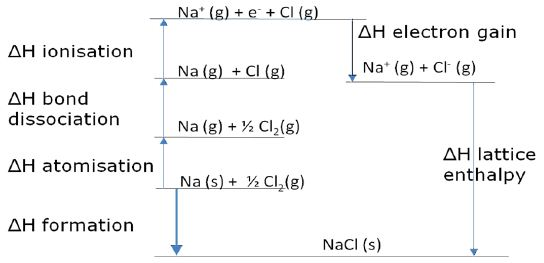Chemistry

# Describe on Born-Haber Cycle

Lattice enthalpies cannot be determined directly but an application of Hess’s law to the formation of an ionic crystal called the ‘Born-Haber cycle’ can be used to calculate the value.

This cycle is an imaginary closed path that includes all the different enthalpy change steps involved in the enthalpy of formation. The steps can be drawn in any order, as long as the positive or negative signs are correct.

Born-Haber Cycle for sodium chloride NaCIHess’s Law states that two alternative routes forming solid crystal Sodium Chloride from the elements Sodium Na and Chlorine Cl will have equal ΔH and as such:

∑ ΔH by ROUTE 1 = ∑ ΔH by ROUTE 2

The above means that if the enthalpy values are known for all the other steps, the lattice enthalpy can be calculated by drawing a Born-Haber cycle.

To successfully calculate enthalpy values one needs to be able to:

i. Draw a Born Haber cycle for any simple (two element) ionic compound.

ii. Write the equations for each of the standard enthalpy change steps.

iii. Calculate any one of the enthalpy changes if given all of the others.